# The mowers

The mowers were to mow two meadows, one twice as big as the other. In the first half of the day, they divided into two equal groups. One continued to mow a larger meadow and cut it all by the end of the day. The second group mowed a smaller meadow but did not mow it all until the end of the day. The mowers cut the rest the next day by one mower for the whole day. How many mowers were there? (We assume that the mowing time in the first and second half of the day was the same, and the mowing power was the same. )

n =  8

### Step-by-step explanation:

S1 = 2•S2
n/2 • 1 + n/2 • (1/2) = S1
n/2 • 1/2 + 1•1 = S2

S1-2S2 = 0
2S1-1.5n = 0
2S2-0.5n = 2

S1 = 6
S2 = 3
n = 8

Our linear equations calculator calculates it.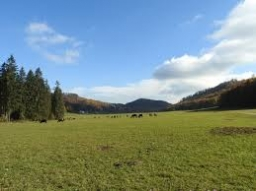Did you find an error or inaccuracy? Feel free to write us. Thank you!Tips to related online calculators
Do you have a linear equation or system of equations and looking for its solution? Or do you have a quadratic equation?
Do you want to convert time units like minutes to seconds?

## Related math problems and questions:

• Lesson exercising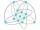The lesson of physical education, pupils are first divided into three groups so that each has the same number. The they redistributed, but into six groups. And again, it was the same number of children in each group. Finally they divided into nine equal g
• Three peopleThree people start doing a work at a same time. The first worked only 2 hours. The second ended 3 hours before the end. On an individual basis, it would take the first time to do the work 10 hours, second 12 hours and 15 hours third. How many hours did it
• Four families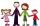Four families were on a joint trip. In the first family, there were three siblings, namely Alica, Betka and Cyril. In the second family were four siblings, namely David, Erik, Filip and Gabika. In the third family, there were two siblings, namely Hugo and
• Two workers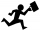One worker needs 40 hours to do a job, and the second would do it in 30 hours. They worked together for several hours, then the second was recalled, and the first completed the job itself in 5 hours. How many hours did they work together, and how much did
• Part-time workers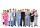On Saturday, 210 part-time workers arrived in the village. One hundred five part-time workers worked on the first field, 60 on the second. The other part-time workers worked in the third field. They all worked in equal groups. How many workers worked in e
• 1200 screws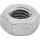1200 screws are to be divided into 3 groups so that in the 1st group there are 300 screws more than in the 2nd group and in the 2nd group 150 screws less than in the 3rd group. How many screws will be in each group?
• Bricklayers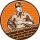The first bricklayer would build a wall in 3 days, the second in 4 days. The first bricklayer worked alone for a day, then the second bricklayer came to his aid and they finished the work together. How long did it take to build the wall?
• The coal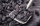The coal stock would be enough to heat a larger room for 12 weeks, a smaller one for 18 weeks. It was heated for four weeks in both rooms, then only in a smaller one. How long was the coal stock enough?
• Two trainsThrough the bridge, long l = 240m, the train passes through the constant speed at time t1 = 21s. A train running along the traffic lights at the edge of the bridge passes the same speed at t2 = 9s. a) What speed v did the train go? b) How long did it take
• Poisson distribution - daisies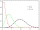The meadow behind FLD was divided into 100 equally large parts. Subsequently, it was found that there were no daisies in ten of these parts. Estimate the total number of daisies in the meadow. Assume that daisies are randomly distributed in the meadow.
• Mason with assistantMason had to complete the work in 10 days. Two and a half days later, his assistant arrived. Together they completed the work in the next three and a half days. How many days would he need an assistant for the same work?
• Panel house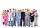The construction company has two groups of workers. Group A has more members than B. Panel house was insulated by group A in 10 days. They insulated the same block of flats together in 6 days. How many days would group B block insulate?
• Painters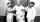One painter could paint a school for seven days. The second one does it for six days. It was necessary to paint the school in two days. The next painter was invited, and all worked together. How long would this painter paint the school itself?
• Digging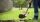The first worker would dig a trench in 6 hours. For the second, the same work would take 4 hours. How long did it take for the trench to be dug if they worked together?
• Two workersTwo workers should fulfill certain task together for 5 days. If the first worker increased their performance twice and second twice fell, it took them just four days. For how many days would handle the entire task first worker himself?
• Two numbersOne number is by 79 larger than the other. If we divide the larger number by the smaller one, we get the ratio 5 and the remainder 11. Determine both numbers.
• Pet store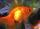In a pet store, they are selling out the fish from one aquarium. Ondra wanted half of all fish, but they don't wish cut by hal fany fish he got one more than demanded. Matthew wished the remaining half of the fish, but as Andrew got half the fish more tha